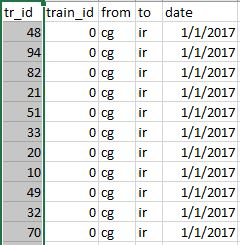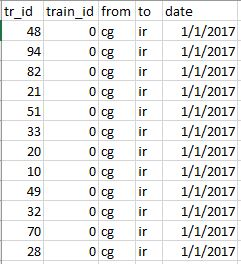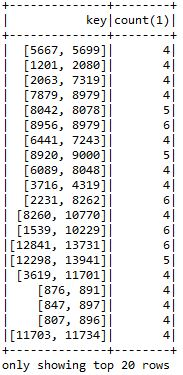# How to proceed taking intersection of sets

Hi,

I am given a data set which contains tr_id (travelers id), train_id (which train ride) all of type integer and unique per traveler and per train ride respectively. I am then asked to determine the travelers who have been on more than 3 rides together.
So the output should look like:

tr_id 1 | tr_id 2 | number of rides together
48 | 54 | 6

My thought process: first delete all travelers that have been on three rides or less
`val window = Window.partitionBy("tr_id")`
` val moreThanThreeRides_df = flight_df.withColumn("count", count("train_id").over(window))`
` .filter(col("count") > 3).drop("count").orderBy("passengerId", "flightId")`

Then I would create a set containing all train_id’s for the corresponding tr_id and map one to the other.
`val collectIds_df = moreThanThreeRides_df.groupBy("tr_id")`
`.agg(collect_set(struct("train_id")).as("Set of Rides")).orderBy("tr_id")`

`````` collectIds_df.withColumn("mapToRides", map(col("tr_id"),col("Set of Rides")))
``````

How I would proceed: intersect the set of flights from one traveler with that of the other traveler, check if it contains more than 3 elements and if so return the respective tr_id’s and number of elements. This would require a nested for loop but the inner loop requires less iterations as (tr_id1,tr_id2) = (tr_id2,tr_id1) as the order does not matter. I have tried to write this down in Scala but without success. How do I write this down?

If there is any better or efficient way of solving this I would be open for that as well. All your help is appreciated!

In Spark you very rarely can use imperative constructs such as a nested for loop. It’s better to think in terms of functional constructs.

I would start by grouping together all travelers that have been on the same train ride and returning all combinations of 2 different travelers. Then you can simply count how many times each combination occurs.

Didn’t try to compile or test this:

``````case class Ride(tr_id: Int, train_id: Int)

val dataset: Dataset[Ride] = ???
dataset
.groupByKey(_.train_id)
.flatMapGroups{ (train_id, rides) =>
rides
.map(_.tr_id)
.toList // let's assume there aren't enough people on 1 train to run out of memory here
.combinations(2)
.map(_.sorted) // make sure tr_id1 is always the smaller one
.map{ case List(tr_id1, tr_id2) => (tr_id1, tr_id2) }
}
.groupByKey(identity)
.count
.filter(_._2 > 3)
``````
1 Like

Can you please share the details, like the columns and sample data? Also, the input of the above mentioned output?

My data looks as followsThe file is ./Train_Data_New.csv
What should I put on val dataset: Dataset[Ride] = ???
I’ve tried to import it as rdd but that doesn’t work. What would you suggest here? Sorry, I’m a bit of a beginner to Scala.

Something like this should do the trick.

``````import sparkSession.implicits._

val dataset: Dataset[Ride] =
sparkSession
.csv("./Train_Data_New.csv")
.select('tr_id cast "int", 'train_id cast "int")
.as[Ride]
``````Here is some sample data. The input of the above output would be the number of times two travelers have at least been on the same ride.

It worked so far! Last question: how do I get the output into the following format:
tr_id1 || tr_id2 || rides together ? I’ve also tried .count.as(“rides together”) but that does not seem to work. I’ve come close!The easiest way is probably using `select` to arrange the columns in your preferred layout. Alternatively you could also map to a custom case class.

``````results.toDF("ids", "rides").select(\$"ids._1" as "tr_id1", \$"ids._2" as "tr_id2", \$"rides")
``````
1 Like

That worked Jasper, you’ve been some great help to me! Thank you so much and all the best to you.